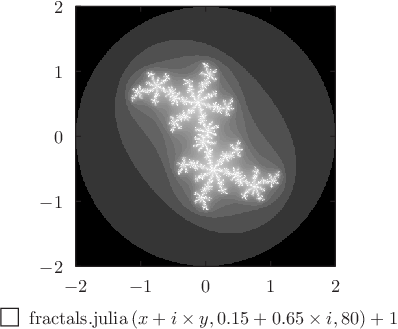# Pyxplot

## Examples - Julia setDownload this example:

Example plot - using the colormap plot style to draw the Julia set

A Julia set, plotted using the colormap plot style.

### Script

```set numerics complex
set sample grid 400x400
set log c1
set nogrid
set xtics 1
set ytics 1
set size square
set key below
set nocolkey
plot [-2:2][-2:2] fractals.julia(x+i*y,0.15+0.65*i,80)+1 with colormap

```